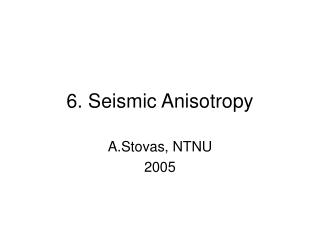# 6. Seismic Anisotropy - PowerPoint PPT PresentationDownload Presentation6. Seismic Anisotropy

6. Seismic AnisotropyDownload Presentation## 6. Seismic Anisotropy

- - - - - - - - - - - - - - - - - - - - - - - - - - - E N D - - - - - - - - - - - - - - - - - - - - - - - - - - -
##### Presentation Transcript

1. 6. Seismic Anisotropy A.Stovas, NTNU 2005

2. Definition (1) • Seismic anisotropy is the dependence of seismic velocity upon angle • This definition yields both P- and S-waves

3. Definition (2) • Saying ”velocity” we mean • ray veocity and wavefront velocity • group velocity and phase velocity • interval velocity and average velocity • NMO velocity and RMS velocity

4. Definition (3) • We have to distinguish between anisotropy and heterogeneity • Heterogeneity is the dependence of physical properties upon position • Heterogeneity on the small scale can appear as seismic anisotropy on the large scale

5. Notations • hi – layer thickness • vi – layer velocity • t0 – vertical traveltime • vP0 – vertical velocity • vNMO – normal moveout velocity • , d, h – anisotropy parameters S2 – heterogeneity coefficient

6. Simple example of anisotropy (two isotropic layers model) isotropic VTI isotropic • hi – layer thickness • vi – layer velocity • t0 – vertical traveltime • vP0 – vertical velocity • vNMO – normal moveout velocity • , d, h – anisotropy parameters S2 – heterogeneity coefficient

7. Elasticity tensor Equation of motion stress Stress-strain relation (Hook’s law) strain The elasticity tensor

8. Symmetry We convert stiffness tensor Cijmn to the stiffness matrix Cab The worst case: Triclinic symmetry 21 different elements The best case: Isotropic symmetry 2 different elements Lame parameters: l and m

9. Seismic anisotropy symmetries Trasverse isotropy symmetry 5 different elements (shales, thin-bed sequences Orthorombicic symmetry 9 different elements (shales, thin-bed sequences with vertical crack-sets)

10. VTI and HTI anisotropy symmetry plane VTI HTI symmetry axis

11. The phase velocities (velocities of plane waves) Cij – stiffness coefficients vi – phase velocity q – phase angle

12. Parametrization (Thomsen, 1984) Vertical velocities Anisotropy parameters

13. Interpretation of anisotropy parameters Isotropy reduction Horizontal propagation

14. Weak anisotropy approximation

15. Weak anisotropy for laminated siltstone

16. Mesaverde shale/sandstone

17. Nonellipticity

18. Wave propagation in homogeneous anisotropic medium Wavefront normal Wavefront tangent k – wavenumber Vgroup – group velocity w – angular frequency vphase – phase velocity p – horizontal slowness Q, q – group and phase angles

19. The anisotropic moveout The hyperbolic moveout (x – offset ot source-receiver separation) The Taylor series coefficient The moveout velocity

20. The anisotropic qP-traveltime in p-domain The horizontal slowness The offset The traveltime Sa – deviation of the slowness squared between VTI and isotropic cases aj – coefficients for expansion in order of slowness

21. The anisotropic traveltime parameters The P-wave The vertical Vp-Vs ratio The S-wave

22. The moveout velocity The critical slowness

23. The velocity moments v0= a0

24. The heterogeneity coefficients

25. The Taylor series The normalized offset squared

26. The traveltime approximations Shifted hyperbola Continued fraction

27. The continued fraction approximations Tsvankin-Thomsen Correct Ursin-Stovas

28. The heterogeneity coefficient S2 Alkhalifah and Tsvankin: Ursin and Stovas: S2 (Ursin and Stovas) reduces to S2 (Alkhalifah and Tsvankin) if g 0is large (acoustic approximation)

29. The traveltime approximations(single VTI layer) Bold – two terms Taylor Empty circles – shifted hyperbola Filled circles – Tsvankin-Thomsen Empty stars – Stovas-Ursin

30. The traveltime approximations(stack of VTI layers)

31. Layering against anisotropy

32. VNMO for dipping reflector a is the angle for dipping reflector Tsvankin, 1995

33. VTI DMO operator Operator shape depends on the sign of h Stovas, 2002

34. If we ignore anisotropy in post-stack time migration • Dipping reflectors are mispositioned laterally. Mislocation is a function of: - magnitude of the average h foroverburden - dip of the reflector - thickness of anisotropic overburden • Diffractions are not completely collapsed, leaving diffraction tails, etc. Alkhalifah and Larner, 1994

35. Determining d • Use VP-NMO from well-log • The residual moveout gives d

36. Dix-type equations (1) Ursin and Stovas, 2004

37. Wave propagation in VTI medium Uz and Ur are transformed verical and horizontal displacement components; Sz and Sr are transformed vertical and horizontal stress components Stovas and Ursin, 2003

38. Up- and down-wave decomposition With linear transformation qa and qb are verical slownesses for P- and S-wave

39. The transformation matrix with the symmetries

40. Scattering matrices Symmetry relations

41. The vertical slowness The vertical slownesses squared are the eigenvalues of the matrix and are found by solving the characteristic equation

42. The R/T coefficients with where superscripts (1) and (2) denote the upper and lower medium, respectively

43. The weak-contrast R/T coefficients q is the vertical slowness

44. The weak-contrast R/T coefficients(Rueger, 1996) m is shear wave bulk modulus:

45. Parametrization • Stiffness coefficients • Velocities • Impedances • Mixed

46. Effect of anisotropy

47. Different parametrizations

48. Contribution from the contrasts

49. Second-order R/T